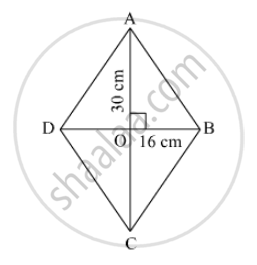SSC (English Medium) Class 8Maharashtra State Board
Share

# If Length of a Diagonal of a Rhombus is 30 Cm and Its Area is 240 Sq Cm, Find Its Perimeter. - SSC (English Medium) Class 8 - Mathematics

#### Question

If length of a diagonal of a rhombus is 30 cm and its area is 240 sq cm, find its perimeter.

#### SolutionLet the other diagonal be d cm
Area of a rhombus = 1/2 (product of diagnols)

⇒ 240 = 1/2 xx (30 x d)

⇒ d = (240 xx 2)/30 = 16

AC = 30 cm
DB = 16 cm
Diagonals of a rhombus bisect at right angles.
In Δ AOB,

AO² + OB² = AB²

⇒ 15² + 8² = AB²

⇒ AB² = 225 + 64 = 289

⇒ AB = √289 = 17 cm

Thus, the side of the rhombus = 17 cm
Perimeter = 4 xx 17 = 68 cm

Is there an error in this question or solution?

#### APPEARS IN

Balbharati Solution for Balbharati Class 8 Mathematics (2019 to Current)
Chapter 15: Area
Practice Set 15.2 | Q: 4 | Page no. 97
Solution If Length of a Diagonal of a Rhombus is 30 Cm and Its Area is 240 Sq Cm, Find Its Perimeter. Concept: Area of a Rhombus.
S